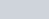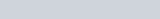To draw a pair f tangents to a circle which are inclined
Question:

To draw a pair f tangents to a circle which are inclined to each other at an angle of 100°, It is required to draw tangents at end points of those two radii of the circle, the angle between which should be:

(a) 100°

(b) 50°

(c) 80°

(d) 200°

Solution:

Given a pair of tangents to a circle inclined to each other at angle of 100°

We have to find the angle between two radii of circle joining the end points of tangents that is we have to findin below figure.Let be the center of the given circle

Let AB and AC be the two tangents to the given circle drawn from point A

Therefore BAC = 100°

Now OB and OC represent the radii of the circle

Therefore[Since Radius of a circle is perpendicular to tangent]

We know that sum of angles of a quadrilateral = 360°

$\angle B A C+\angle A C O+\angle C O B+\angle O B A=360^{\circ}$
$100^{\circ}+90^{\circ}+\angle C O B+90^{\circ}=360^{\circ}$
$\angle C O B=360^{\circ}-280^{\circ}$
$\angle C O B=80^{\circ}$